# Remove Last Character(s) From Right – Excel & Google Sheets

This tutorial will demonstrate how to remove the last character(s) from a string of text in Excel and Google Sheets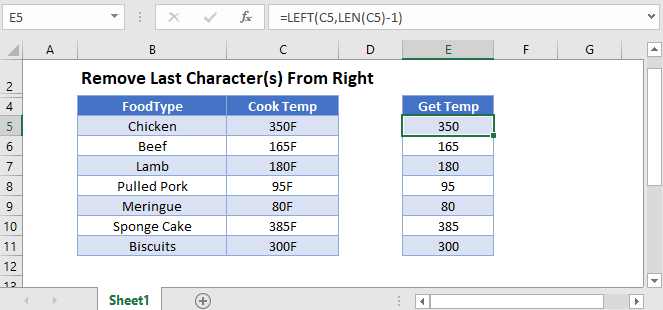## Remove Characters From the Right

To remove characters from the right of a cell, we can use the LEFT Function to return a certain number of characters from the left and the LEN Function to count how many characters are in the cell, allowing us to remove n (ex. 1) characters from the right:

``=LEFT(C3,LEN(C3)-1)``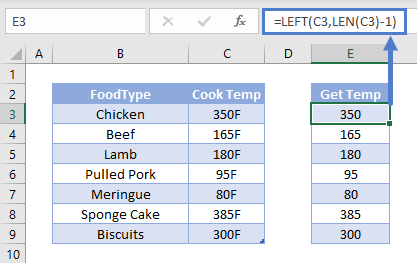We will walkthrough this below.

## LEN Function – Count Characters in a Cell

First, we will use the LEN Function to count the number of characters in the cell:

``=LEN(C3)``In the next section, we will use the number of characters to trim off a certain number of characters from the right.

## LEFT Function – Show Characters from the Left

The LEFT Function returns a certain number of characters from the left side of a cell. For the number of characters, we will use the result of the LEN Function minus the number of characters to remove (ex. 1):

``=LEFT(C3, E3-1)``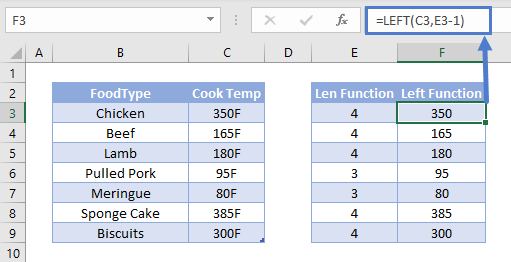Combining these functions yields the original formula.

``=LEFT(C3,LEN(C3)-1)``## Split Numbers and Text

You may be trying to split numbers from text. When you split numbers, you should use the VALUE Function to convert the number stored as text to a number.

``=VALUE(LEFT(C3,LEN(C3)-1)``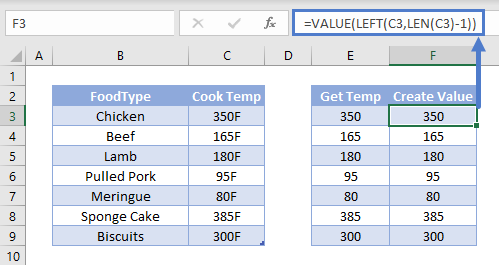Converting numbers stored as text to numbers is necessary for Excel to treat the numbers as numbers.

## Remove Last Character(s) From Right in Google Sheets

All the examples explained above work the same in Google sheets as they do in Excel.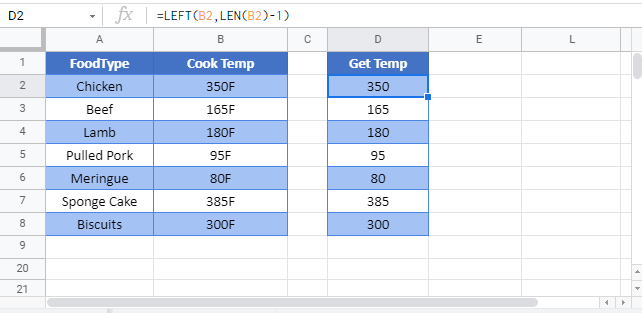### Excel Practice Worksheet

Practice Excel functions and formulas with our 100% free practice worksheets!